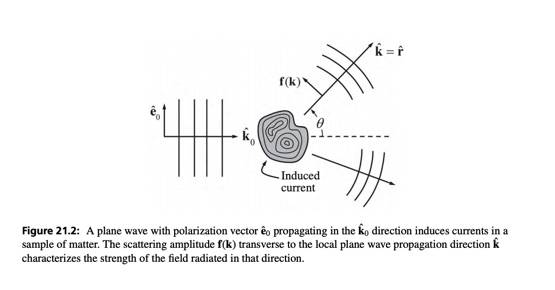# Rayleigh Scattering Conceptual Question about the Color of the Sky

PhDeezNutz
Homework Statement:
Why when we look at the sun directly is it red/orange? and why does the sky appear blue?
Relevant Equations:
For Rayleigh Scattering we have the scattering cross section

##\frac{\ d \sigma}{d \Omega} = \left( \frac{k_0^2 \alpha}{4 \pi}\right)^2 \left( 1 - \left|\hat{r} \cdot \hat{e}_0 \right|^2 \right)##

Where ##\hat{r} = \hat{k}## (the scattered wave propagates radially). ##\hat{e}_0## is the unit vector of the incident electric field. For the sake of simplicity I'm going to assume normal incidence (see picture below)

The ##k_0^4## dependence in the numerator implies a ##\frac{1}{\lambda^4}## dependence of ##\frac{d \sigma}{d \Omega}##.We know ##\lambda_{blue} < \lambda_{red}## so citing the formula above (in the relevant equations) it's apparent that blue light is scattered more than red light. But presumably this would hold for ##\theta = 0## as well but when we look at the sun head on we don't see blue, we see red/orange.

Am i interpreting this formula wrong?

Last edited by a moderator:
•JD_PM

PhDeezNutz
I think it's because of the following. Even when ##\theta = 0## blue light is scattered more and red light is scattered less and that's why the "inner part" is red and the "outer part" is blue.

I think it's just that simple.

Staff Emeritus
Homework Helper
What do you mean by inner part and outer part?

•PhDeezNutz
PhDeezNutz
What do you mean by inner part and outer part?

inner part - apparent angular size of the sun

outer part - the rest of the blue sky

Staff Emeritus
Homework Helper
Your answer feels incomplete to me. In the first post, you said blue light is scattered more than red light in the forward direction and asked why this didn't mean the Sun appeared blue. In the second post, you said blue light is scattered more than red light in the forward direction and concluded this is why the Sun appears red. I don't really see an explanation in there.

•PhDeezNutz
PhDeezNutz
Your answer feels incomplete to me. In the first post, you said blue light is scattered more than red light in the forward direction and asked why this didn't mean the Sun appeared blue. In the second post, you said blue light is scattered more than red light in the forward direction and concluded this is why the Sun appears red. I don't really see an explanation in there.

yeah I think I was trying to reconcile it anyway I could...and it fell short. Still open to suggestions.

Staff Emeritus
Homework Helper
It might be easier to think about the total cross section, ##\sigma = \int (d\sigma/d\Omega)\,d\Omega##. You can interpret ##\sigma_{\rm blue} > \sigma_{\rm red}## to mean that the air molecules are a "bigger" target than for red light. In other words, the probability of an interaction between the light and an air molecule is greater for blue light than for red light.

Now think of light along your line of sight coming from the Sun to your eyes. For simplicity, let's assume equal amounts of blue and red light are emitted by the Sun. If you were on the Moon, equal amounts of blue and red light would reach your eyes because there's nothing that gets in the way between you and the Sun. If you're on the Earth, however, the atmosphere is there. What's going to happen?

•PhDeezNutz
Homework Helper
2022 Award
Not all the "forward" light is scattered. The stuff that is not scattered goes on through but diminished preferentially of its blue light. If you work it out, the total scattering of sunlight is about 10-5 per meter of air at sea level density. Depending on your interest you could calculate the total Rayleigh cross section (per atom) and figure what percentage of the sunlight is Rayleigh scattered. Of course the path gets longer as the sun sets but even at dusk it is only a fraction.

•PhDeezNutz
Homework Helper
Gold Member
A good follow up question is: why doesn't the sky appear purple.

•PhDeezNutz and JD_PM
JD_PM
A good follow up question is: why doesn't the sky appear purple.

That's an interesting question.

Based on the Rayleigh scattering cross-section formula

$$\sigma_s \sim \frac{1}{\lambda^4}$$

We indeed have ##\sigma_{violet} > \sigma_{blue}## so one would a priori expect to see the sky purple. The answer has to do with how we perceive violet

•etotheipi and PhDeezNutz